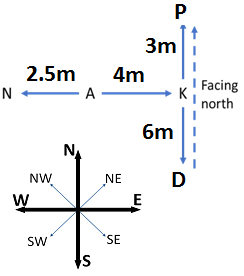# Direction Sense Test - Logical Reasoning (MCQ) Questions for Q. 30021

Q.  Study the information given below carefully, and answer the question that follow:

On a stage, D, N, A and P are standing as described below facing North.
1) N is 2.5 m to the west of A.
2) K is 4 m to the right of A.
3) D is 6 m to the south of K.
4) P is 9 m to the north of D.

If a boy walks from N, meets A followed by K, D and then P, how many metres has he walked if he has travelled the straight distance all through?

- Published on 15 May 17

a. 15 m
b. 18 m
c. 21.5 m
d. 22.5 m

Required distance = NA + AK + KD + DP = 2.5 + 4 + 6 + (6 + 3) = 21.5 m6

#### Discussion

• ATUL BHARTI   -Posted on 03 Feb 21
amazing explain dude great way to explain this questions
• gg   -Posted on 07 Jun 19
67yghuygh

## ➨ Post your comment / Share knowledgeEnter the code shown above:

(Note: If you cannot read the numbers in the above image, reload the page to generate a new one.)